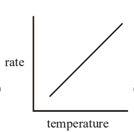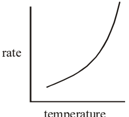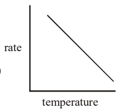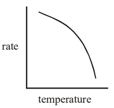The rate of a reaction increases 4-fold when when concentration of reactant is incresed 16 times. If the rate of reaction is when concentration of the reactant is $4×{10}^{-4}$, the rate constant of the reaction will be

(A) $2×{10}^{-4}mol{e}^{1/2}{L}^{-1/2}{S}^{-1}$

(B) $1×{10}^{-2}{S}^{-1}$

(C) $2×{10}^{-4}mol{e}^{-1/2}{L}^{-1/2}{S}^{-1}$

(D)

High Yielding Test Series + Question Bank - NEET 2020

Difficulty Level:

If ‘a’ is the initial concentration of a substance which reacts according to zero order kinetic and k is rate constant, the time for the reaction to go to completion is-

(A) a/k

(B) 2/ka

(C) k/a

(D) Infinite

High Yielding Test Series + Question Bank - NEET 2020

Difficulty Level:

The following data are obtained from the decomposition of a gaseous compound

Initial pressure, atm               1.6       0.8       0.4

Time for 50% reaction, min     80       113      160

The order of the reaction is

(A) 1.0

(B) 1.5

(C) 2.0

(D) 0.5

High Yielding Test Series + Question Bank - NEET 2020

Difficulty Level:

For a given reaction the concentration of the reactant plotted against time gave a straight line with negative slope.

The order of the reaction is-

(A) 3

(B) 2

(C) 1

(D) 0

High Yielding Test Series + Question Bank - NEET 2020

Difficulty Level:

The rate constant of a first order reaction is generally determined from a plot of

(A) Concentration of reactant vs time t

(B) log (concentration of reactant) vs time t

(C)

(D) Concentration of reactant vs log time t

High Yielding Test Series + Question Bank - NEET 2020

Difficulty Level:

Which of the following correctly represents the variation of the rate of the reaction with temperature ?

(A)(B)(C)(D)High Yielding Test Series + Question Bank - NEET 2020

Difficulty Level:

The plot of log k versus $\frac{1}{T}$is linear with a slope of

(A) $\frac{{E}_{a}}{R}$

(B) $\frac{-{E}_{a}}{R}$

(C) $\frac{{E}_{a}}{2.303R}$

(D) $\frac{-{E}_{a}}{2.303R}$

High Yielding Test Series + Question Bank - NEET 2020

Difficulty Level:

If a reaction A + B$\to$ C is exothermic to the extent of 30 kJ/mol and the forward reaction has an activation energy 70 kJ/mol, the activation energy for the reverse reaction is

(A) 30 kJ/mol

(B) 40kJ/mol

(C) 70 kJ/mol

(D) 100 kJ/mol

High Yielding Test Series + Question Bank - NEET 2020

Difficulty Level:

A first order reaction takes 40 min for 30% decomposition.Calculate ${t}_{1/2}$

(A) 77.7 min.

(B) 27.2 min.

(C) 55.3 min.

(D) 67.3 min.

Concept Questions :-

First Order Reaction Kinetics
High Yielding Test Series + Question Bank - NEET 2020

Difficulty Level:

For a certain reaction involving a single reactant, it is found that ${C}_{0}\sqrt{T}$ is constant where ${C}_{0}$is the initial concentration of the reactant and T is the half-life. What is the order of the reaction ?

(A) 1

(B) Zero

(C) 2

(D) 3

Concept Questions :-

Order and Molecularity